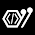## Friday, March 1, 2019learn c++ programming

like function overloading, we also overload the constructor. we can use the one or more constructor in our program with different parameters. and this feature is called constructor overloading.

Example:
class test
{
public:

test();               //constructor with no parameter
test(int x);       // constructor with one parameter
test(int x, int y); // constructor with two parameter.

};

#include <iostream>

class test
{
int a,b;

public:

test()     //define first costructor with no parameter
{
a=0;
b=0;
std::cout<<"\n value of a and b is: "<<a<< b;
}

test(int x) //define second constructor with one parameter
{
a=x;
b=x;
std::cout<<"\n value of a and b is: "<<a<< b;
}
test(int x, int y) //define third constructor with second parameter
{
a=x;
b=y;
std::cout<<"\n value of a and b is "<<a<< b;
}

};

int main()
{
test t1; //called first constructor
test t2(10); //called second constructor
test t3(10,20); //called third constructor

return 0;
}learn c++ programming
feel free to use this code. just copy this code and paste in your compiler or editor and use it.

1.